# PG API Examples - 2020.2 English

Document ID
UG958
Release Date
2020-11-18
Version
2020.2 English

## Hello World

In this example, you will run the PG API in the learning mode where you can type the commands in the MATLAB® command shell.

1. To start a new learning session, in MATLAB command console, run: `xBlock`.
2. Type the following three commands in MATLAB command console to create a new Subsystem named '`Subsystem`' inside a new model named '`untitled'`.
``````
[a, b] = xInport('a', 'b');
s = xOutport('s');
``````
Figure 1. Subsystem Example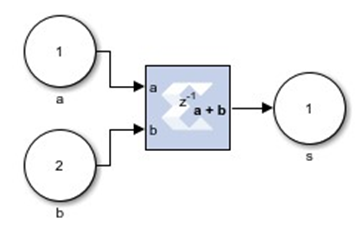The above commands create the Subsystem with two Simulink Inports `a` and `b`, an adder block having a latency of one, and a Simulink Outport `s`. The two Inports source the adder which in turn sources the Subsystem outport. The `AddSub` parameter refers to the AddSub block inside the xbsIndex_r4 library. By default, if the full block path is not specified, `xBlock` will search `xbsIndex_r4` and built-in libraries in turn. The library must be loaded before using `xBlock`. So please use `load_system` to load the library before invoking `xBlock`.

Tip: If you type `adder` in the MATLAB console, System Generator will print a brief description of the adder block to the MATLAB console and the block is highlighted in the Simulink diagram. Similarly, you can type `a`, `b`, and `s` to highlight Subsystem Inports and Outports.

## MACC

1. Run this example in the learning mode. To start a new learning session, run: `xBlock`.
2. Type the following commands in the MATLAB console window to create a multiply-accumulate function in a new Subsystem.
``````
[a, b] = xInport('a', 'b');
mac = xOutport('mac');
m = xSignal;
mult = xBlock('Mult', struct('latency', 0, 'use_behavioral_HDL', 'on'), {a, b},
{m});
acc = xBlock('Accumulator', struct('rst', 'off', 'use_behavioral_HDL', 'on'), {m},
{mac});
``````

By directing System Generator to generate behavioral HDL, the two blocks should be packed into a single DSP48 block. As of this writing, Vivado synthesis only does so if you force the multiplier block to be combinational.

Figure 2. Forcing a Mult Block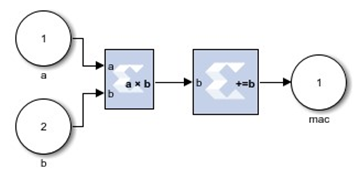Note: If you do not close the model that is created in example 1, example 2 is created in a model named `untiltled1`. Otherwise, a new model, `untitled`, is created for this example.
Tip: The PG API provides functions to get information about blocks and signals in the generated Subsystem. After each of the following commands, observe the output in the MATLAB console and the effect on the Simulink diagram.
``````
mult_ins = mult.getInSignals
mult_ins{1}
mult_ins{2}
src_a = mult_ins{1}.getSrc
src_a{1}
m_dst = m.getDst
m_dst{1}
m_dst{1}.block

``````

## MACC in a Masked Subsystem

If you want a particular Subsystem to be generated by the PG API and pass parameters from the mask parameters of that Subsystem to PG API, you need to run the PG API in production mode, where you need to have a physical M-function file and pass that function to the `xBlock` constructor.

1. First create the top-level PG API M-function file `MACC_sub.m` with the following lines.
``````
function MACC_sub(latency, nbits)
[a, b] = xInport('a', 'b');
mac = xOutport('mac');
if latency <= 0
error('latency must be positive');
elseif latency == 1
a_in = a; b_in = b;
else
[a_in, b_in] = xSignal;
dblock1 = xBlock('Delay', struct('latency', latency - 1, 'reg_retiming', 'on'),
{a}, {a_in});
block2 = xBlock('Delay', struct('latency', latency - 1, 'reg_retiming', 'on'),
{b}, {b_in});
end
m = xSignal;
mult = xBlock('Mult', struct('latency', 0, 'use_behavioral_HDL', 'on'), {a_in,
b_in}, {m});
acc = xBlock('Accumulator', struct('rst', 'off', 'n_bits', nbits,
'use_behavioral_HDL', 'on'), {m}, {mac});

``````
Figure 3. Top-Level PG API M-Function File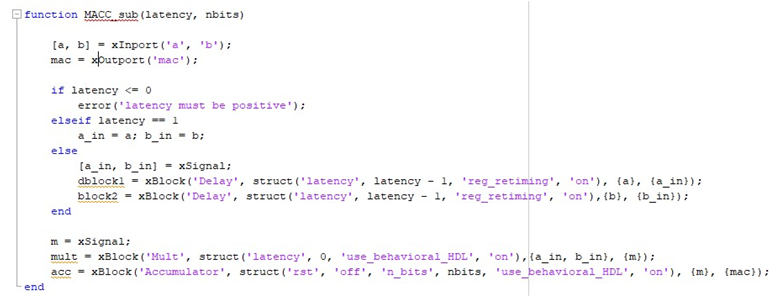Figure 4. Adding Latency Parameter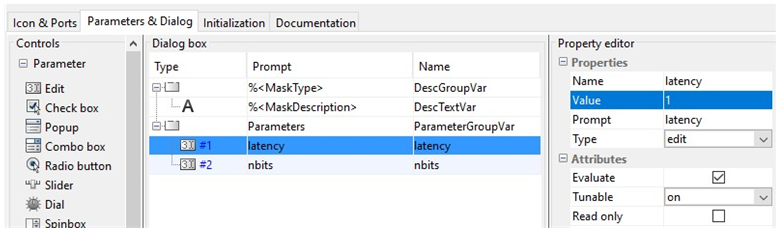3. Then put the following lines to the mask initialization of the Subsystem.
``````
config.source = str2func('MACC_sub');
config.toplevel = gcb;
xBlock(config, {latency, nbits});
``````

In the production mode, the first argument of the `xBlock` constructor is a MATLAB struct for configuration, which must have a source field and a toplevel field. The source field is a function pointer pointing to the M-function and the toplevel is a string specifying the Simulink Subsystem. If the top-level field is 1, an untitled model is created and a Subsystem inside that model is created.

Figure 5. Adding nbits Parameter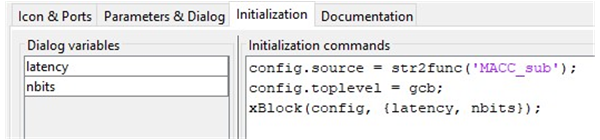Alternatively you can use the MATLAB struct call to create the toplevel configuration:

``````
xBlock(struct('source', str2func(MACC_sub), 'toplevel', gcb),{latency,

nbits});
``````

Then click OK.

You'll get the following Subsystem.

Figure 6. Creating Toplevel Configuration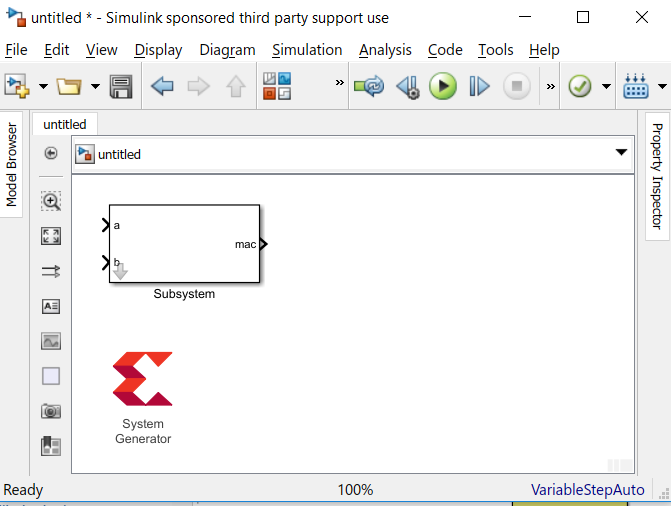4. Set the mask parameters as shown in the following figure, then click OK:
Figure 7. Adding Mask Parameters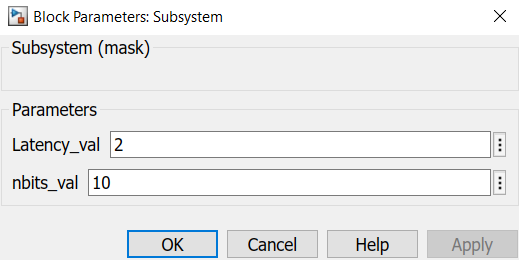The following diagram is generated:

Figure 8. Generated Diagram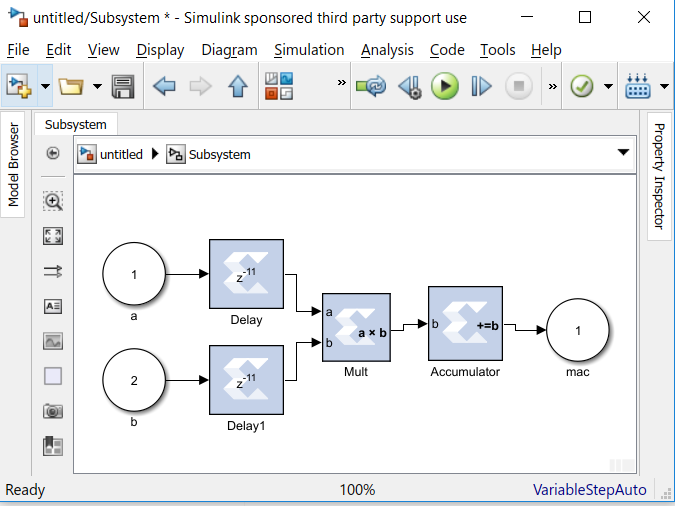Tip: Open `MACC_sub.m` in the MATLAB editor to debug the function. By default the `xBlock` constructor will do an auto layout in the end. If you want to see the auto layout every time a block is added, invoke the toplevel `xBlock` as the following:
``````
config.source = str2func('MACC_sub');
config.toplevel = gcb;
config.debug = 1;
xBlock(config, {latency, nbits});
``````

By setting the debug field of the configuration struct to be 1, you run the PG API in debug mode where every action will trigger an auto layout.

Tip: Most often you only want to re-generate the Subsystem if needed. The `xBlock` constructor has a caching mechanism. You can specify the list of dependent files in a cell array, and set the `depend` field of the toplevel configuration with this list. If any file in the 'depend' list is changed, or the argument list that passed to the toplevel function is changed, the Subsystem is re-generated. If you want to have the caching capability for the MACC_sub, invoke the toplevel xBlock as the following:
``````
config.source = str2func('MACC_sub');
config.toplevel = gcb;
config.depend = {'MACC_sub.m'};
xBlock(config, {latency, nbits});
``````

The depend field of the configuration struct is a cell array. Each element of the array is a file name. You can put a p-file name or an M-file name. You can also put a name without a suffix. The `xBlock` will use the first in the path.